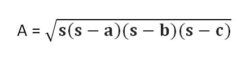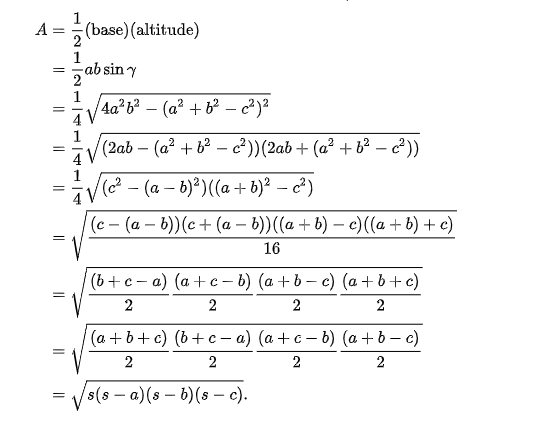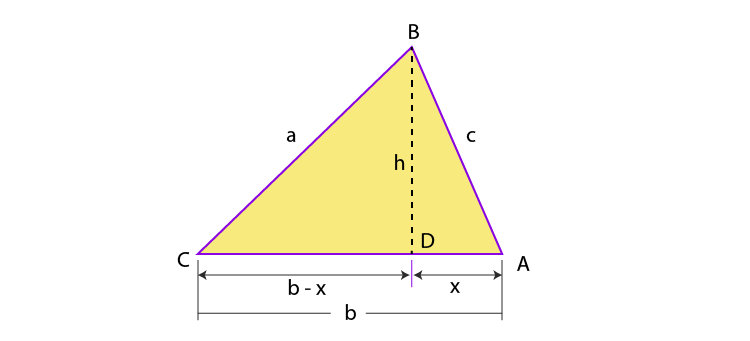# Heron's Formula

Heron’s formula is one of the most important concepts used to find the area of a triangle when all the sides are known. Hero of Alexandra was a great mathematician who derived the formula for the calculation of the area of a triangle using the length of all three sides. It is also termed as Hero’s Formula. He also extended this idea to find the area of quadrilateral and also higher-order polygons. This formula has its huge applications in trigonometry such as proving the law of cosines or law of cotangents, etc. This is one of the important topics in class 9 chapter 12.

## Heron’s or Hero’s Formula

According to Heron, we can find the area of any given triangle, whether it is a scalene, isosceles or equilateral, by using the formula, provided the sides of the triangle. Suppose, a triangle ABC, whose sides are a, b and c, respectively. Thus, the area of a triangle can be given by;Where S is semi-perimeter = (a+b+c) / 2

And a, b, c are the three sides of the triangle.

Example: A triangle PQR has sides a=4, b=13 and c=15. Find the area of the triangle.

Semiperimeter of triangle PQR, s = (4+13+15)/2 = 32/2 = 16

By heron’s formula, we know;

A = √[s(s-a)(s-b)(s-c)

Hence, A = √[16(16-4)(16-13)(16-15) = √(16 x 12 x 3 x 1) = √576 = 24

This formula is applicable to all types of triangles. Now let us derive the area formula given by Heron.

## Derivation of Heron’s Formula

There are two methods by which we can derive the Hero’s formula. First by using trigonometric identities and cosine rule. Secondly by the solving algebraic expression using Pythagoras theorem. Let us see one by one both the proofs or derivation.

### Proof using Trigonometric Cosine Rule

Let us prove the result using the law of cosines:

Let a, b, c be the sides of the triangle and α, β, γ are opposite angles to the sides.

We know that,  the law of cosines is

$Cos \gamma =\frac{a^2+b^2-c^2}{2ab}$

Again, using trig identity, we have

$Sin \gamma = \sqrt{1-cos^2γ}$

= $\frac{4a^2b^2-(a^2+b^2-c^2)^2}{2ab}$

Here, Base of triangle = a

Altitude = b sinγ

Now,### Proof Using Pythagoras Theorem

Area of a Triangle with 3 Sides

Area of ∆ABC is given byA = 1/2 bh _ _ _ _ (i)

Draw a perpendicular BD on AC

x2 + h2 = c2

x2 = c2 − h2—(ii)

⇒x = √(c2−h2)−−−−−−—(iii)

Consider a ∆CDB,

(b−x)2 + h2 = a2

(b−x)2 = a2 − h2

b2 − 2bx + x2 = a2–h2

Substituting the value of x and x2 from equation (ii) and (iii), we get

b2 – 2b√(c2−h2)+ c2−h2 = a2 − h2

b2 + c2 − a2 = 2b√(c2 − h2)

Squaring on both sides, we get;

(b2+c2–a2)2 = 4b2(c2−h2)

$\frac{(b^2 + c^2 -a^2 )^2}{4b^2} = c^2 – h^2$

$h^2~ = ~c^2 ~-~\frac{(b^2 +c^2 -a^2 )^2}{4b^2}$

$h^2~ =~\frac{4b^2 c^2 – (b^2 +c^2 -a^2 )^2 }{4b^2}$

$h^2~=~\frac{(2bc)^2 -(b^2+c^2- a^2 )^2}{4b^2}$

$h^2~=~\frac{[2bc+(b^2 +c^2 -a^2 )][2bc-(b^2+c^2-a^2)]} {4b^2}$

$h^2~ =~\frac{[(b^2+2bc+c^2)-a^2][a^2-(b^2- 2bc+c^2)]}{4b^2}$

$h^2~=~\frac{[(b+c)^2 – a^2 ].[a^2 -(b-c)^2 ]}{4b^2}$

$h^2~=~\frac{[(b+c)+a][(b+c)-a].[a+(b-c)][a-(b-c)]}{4b^2}$

$h^2~=~\frac{(a+b+c)(b+c-a)(a+c-b)(a+b-c)}{4b^2}$

The perimeter of a ∆ABC is

P= a+b+c

$⇒~h^2~ =~\frac{P(P – 2a)(P – 2b)(P -2c)}{4b^2}$

$⇒~h~ = \sqrt{P(P – 2a)(P – 2b)(P -2c)}{2b}$

Substituting the value of h in equation (i), we get;

$A~=~\frac{1}{2} b \frac{\sqrt{P(P – 2a)(P – 2b)(P -2c)}}{2b}$

$A~=~\frac{1}{4}\sqrt{(P(P – 2a)(P – 2b)(P -2c)}$

$A~=~\sqrt{\frac{1}{16} P(P – 2a)(P – 2b)(P -2c)}$

$A~=~\sqrt{\frac{P}{2}\left(\frac{P – 2a}{2}\right)\left(\frac{P – 2b}{2}\right)\left(\frac{P -2c}{2}\right)}$

Semi perimeter(S) = $\frac{perimeter}{2}~=~\frac{P}{2}$

$⇒~A~=~\sqrt{S(S – a)(S – b)(S – c)}$

Note: Heron’s formula is applicable to all type of triangles and the formula can also be derived using the law of cosines and the law of Cotangents.

### Heron’s Formula Problems

Let us now look into some example to have a brief insight into the topic:

Example 1: Find the area of a trapezium, length of whose parallel sides is given as 22cm and 12 cm and the length of other sides are 14 cm each.

Solution: Let PQRS be the given trapezium in which PQ= 22cm, SR= 12cm,

PS=QR=14cm.

Constructions: Draw OR||PS

Now, PORS is a parallelogram in which PS||OR and PO||SR

Therefore, PO=SR=12cm

⇒OQ = PQ-PO = 22 -12 = 10cm

In ∆OQR , we have

$S~ = ~\frac{14+14+10}{2}~ =~\frac{38}{2}~=~19$

Area of ∆OQR = $\sqrt{S(S – a)(S – b)(S –C)}$

= $\sqrt{ (19(19 – 14)(19 – 14)(19 –10))}$

= $\sqrt{4275}$

= $15 \sqrt{19} cm^2$ ………(i)

We know that Area = $\frac{1}{2} \times b \times h$

$\Rightarrow 15\sqrt{19} = \frac{1}{2} \times 10 \times h$

$\Rightarrow h = 5\sqrt{19}$ …………..(ii)

Area of trapezium = $\frac{1}{2}~ (PQ+SR) × h$

= $\frac{1}{2}(22+12) × 3 \sqrt{19}$

= $51 \sqrt{19} cm^2$

Example 2: Find the area of the triangle whose sides measure 10 cm, 17 cm and 21 cm.  Also determine the length of the altitude on the side which measures 17 cm?

Solution: S = $\frac{a+b+c}{2}$ = $\frac{10+17+21}{2}$ = 24

Area of Triangle= $\sqrt{s(s-a)(s-b)(s-c)}$

$\sqrt{24 \times 14 \times 7 \times 3}$

$\sqrt{7056}$ = 84 square cm

Taking 17 cm as the base length we need to find the height

Area, A = 1/2 x base x height

1/2  x 17 x h = 84   or h = 168/17 = 9.88 cm   (Rounded to the nearest hundredth).

To solve more problems based on Heron’s Formula, Register with BYJU’S today and clear your all doubts on maths concepts.  We provide detailed and step by step solutions to all questions asked by you. Also, take free tests to practice for exams.

#### 1 Comment

1. Swapnes Barik

very nice